## XmR

XmR is a moving range chart. It is used when only samples of size one are available. The moving range statistic is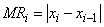The control limits are computed using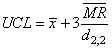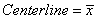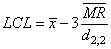Where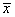is the mean of the observations,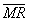is the mean of the moving ranges and is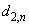is the mean of the distribution of the ranges of n samples from the normal dis­tribution with mean zero and standard deviation one.

### XmR Example

The viscosity of aircraft primer paint was measured. Since the paint is produced in batches, only single samples are available (Montgomery 251).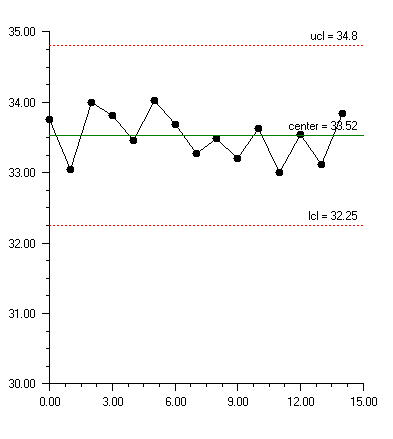using Imsl.Chart2D;

using Imsl.Chart2D.QC;

public class SampleXmR : FrameChart {

static double[] viscosity = {

33.75, 33.05, 34.00, 33.81, 33.46, 34.02, 33.68,

33.27, 33.49, 33.20, 33.62, 33.00, 33.54, 33.12, 33.84

};

public SampleXmR() {

Chart chart = this.Chart;

AxisXY axis = new AxisXY(chart);

XmR xmr = new XmR(axis, viscosity);

}

public static void Main(string[] argv) {

System.Windows.Forms.Application.Run(new SampleXmR());

}

}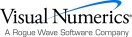Visual Numerics - Developers of IMSL and PV-WAVEhttp://www.vni.com/PHONE: 713.784.3131FAX:713.781.9260